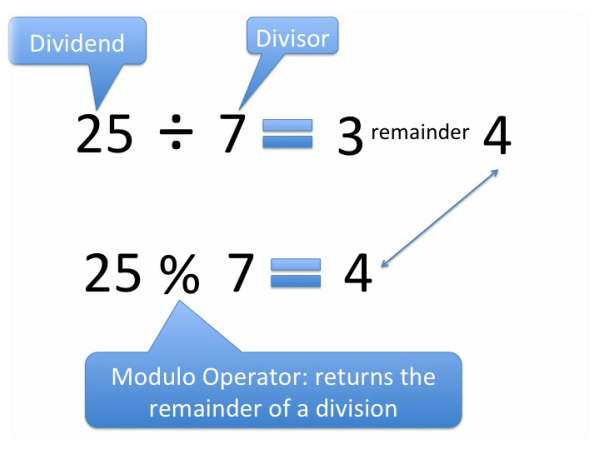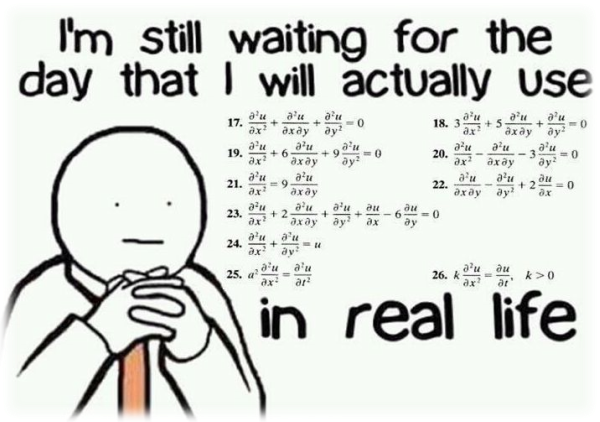# Obviously

After a while had passed, I returned to Ritchie to see about that supposed promotion to Wonderland . The more I thought about it, the more the memories of horror movies arose in my mind; the ones where the main characters are always promised that they'll get to go somewhere great, but in the end, they just end up dead.

That was not going to be my outcome, I told myself. Obviously. I already had a plan to get home, and Wonderland would just be a small diversion. For now, I just needed to lay low, learn some more programming, and wait for Noname to repair everything so that I could get out of here.

"Let's get back to our topics," Ritchie said, pulling me out of my thoughts.

# Modulus

"You already know a lot about math operations, Teo, including operators such as + , - , * , and / . But there is one very important operation that you haven't met yet: the modulus operation. We use a % sign for it. If the operands are integers, modulus returns the remainder of dividing x by y ." Ritchie said."What's a remainder again?" I asked, "It's been a while since I was in math class and..."
"Come on, Teo," Ritchie interrupted. "Maybe you aren't the right fit for the Wonderland promotion." He sighed before continuing. "Ok, listen. Imagine you have 13 candies and 4 equal boxes. Each box must contain 4 candies. How many full boxes can you get?" Ritchie asked.
"3 boxes."
"Correct. And how many candies would not be packed?"
"One candy will be left because you can't have a partially filled box." I replied.

"There you go! This leftover, or 'remainder,' is a result of a modulus operation. 13 modulus 4 will be 1. You can write it this way: 13 % 4 = 1 . That's exactly what you've done inside your brain. Here is a formal definition of the remainder that is returned by the modulus operation of x and y:"
q = x / y
r = x % y
then
x = q * y + r.

"So, in our example: 13 = 3 * 4 + 1."
"We'll only focus on modulus operations with integer types." Ritchie said. "Here is an example:"

``````int result = a % b;
``````

"Ritchie, this seems complicated. When would you actually use this?" I asked.
"The modulus operation is used extensively in programming." Ritchie replied. "For example, say you need to write a program to sort students into classrooms. You have 3 classrooms, and the user enters the number of students as an input to your program. Before going through the trouble of sorting the students, it would be helpful to first check if they can be distributed equally or not by using the modulus operation. Here is an example that I've written:"

``````int studentsNumber = Console.ReadLine();
int notFitCount = studentsNumber % 3;

if (notFitCount == 0)
Console.WriteLine("Can distribute students equally.");
else
Console.WriteLine(\$"Can't distribute students equally.
Students left: {notFitCount}");
``````

"Okay, I see now," I said, "but why in that example do you compare the result of the modulus operation with zero, Ritchie?"
" If the result of a modulus b is zero, it means that a is divisible by b. Sometimes, the power of using the modulus operator is just determining if a number is divisible by another. Divisible means that if a is divided by b , the result is an exact whole number. For example, 12 is divisible by 2, and 6 is divisible by 3. In contrast, 13 is not divisible by 4. Do you follow?" Ritchie asked.
"Sure, I get it." I said.

This first task was easy enough, but my mind was swimming with all this new information. In school, we were taught things around the concept of the modulus ; the remainder , how to divide... but never the modulus itself. In programming, it turns out that modulus is an important operation that helps to understand whether one number is divisible by another. Who would have thought; we spent all that time in school actually finding the remainder, but in the future, we'd be more concerned about whether there was a remainder.
Ritchie proposed a new task while I was silently cursing my school teachers.

"This next task used to be a classic interview question for programmers back in the old days." Ritchie said.

"Now I think you'll actually have a chance at being accepted in Wonderland. Good job, Teo." Ritchie said.
"Thanks," I said, "after all this, I need a serious coffee break." I got up to leave, but Ritchie gestured to stay where I was.
"Not so fast my friend. One more thing before you go. There's a Math class that you should learn.""Is it all of the math in the world in one place?" I joked, but got no response from Ritchie. It either flew over his head or he didn't want to acknowledge it.
"No, but this is a place where the creators of C# gathered a lot of useful math functions. Here's a list of what we use most in the resistance:"

Function Meaning
``````Math.Sin(double)
``````
Returns the sine of the specified angle
``````Math.Cos(double)
``````
Returns the cosine of the specified angle
``````Math.Pow(double, double)
``````
Returns a specified number raised to the specified power
``````Math.Min(int, int)
``````
Returns the smaller of two integers
``````Math.Max(int, int)
``````
Returns the larger of two integers

"And here are some examples of these in use:"

``````Console.WriteLine(Math.Min(2, 3)); // Outputs 2
Console.WriteLine(Math.Max(2, 3)); // Outputs 3
Console.WriteLine(Math.Pow(2.0, 3.0)); // Outputs 8
``````

"You can take a look at the complete list of C# Math functions if you want." Ritchie added.
"Wait, you mean I don't need to write those Min and Max functions myself anymore? They're already written in the Math class by C# creators? Why didn't you tell me!?" I asked angrily.

"We have a rule here at the base. Before using a pre-made function, you need to learn to write your own first. At least at the beginning of your journey. Some day you'll understand why we do that. Starting now, you can use the functions in the Math class instead of writing them by yourself every time."

I was still mad that my time had been wasted, but I could begrudgingly understand the idea of writing a function on my own before using one from an existing class. That way, I would at least know the basics of what the function was doing before blindly letting it do the work for me.At Codeasy we believe that learning should be fun and engaging. Please reach out to us to share your feedback and collaboration ideas.